## A beam of green light is made to pass through two slits that are 5.0 x 10−3 meters apart. On a screen 4.0 meters away from the slits, an int

Question

A beam of green light is made to pass through two slits that are 5.0 x 10−3 meters apart. On a screen 4.0 meters away from the slits, an interference pattern appears with bands of light separated by 4.2 x 10−4 meters. What is the wavelength of the light?

in progress 0
2 months 2021-07-30T23:35:56+00:00 2 Answers 10 views 0

5.3 x 10^−7 m

Explanation:

Significant figures and units are important. 525 nm (as stated in the other answer shown) is technically correct, but the question requires the answer to be in meters, and have two significant figures. Therefore, the answer should be rounded from 5.25 to 5.3, and kept in scientific notation. This gives us the solution 5.3 x 10^−7 m, which should be the third of the selectable options.

The wavelength of light is 525 nm

Explanation:

Given data

Distance between slits, d = 5 x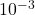m

Distance between slit and screen, D = 4.0 m

band separation, Δx = 4.2 x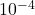m

To find: the wavelength of light, λ = ?

From young’s double slit experiment, we know that the formula to calculate wavelength is as follows:

λ = Δx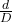Substituting the known values, we get

wavelength of light, λ = (4.2 xx 5 x)/ 4.0

= 5.25 x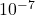m

= 525 x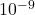m

= 525 nm